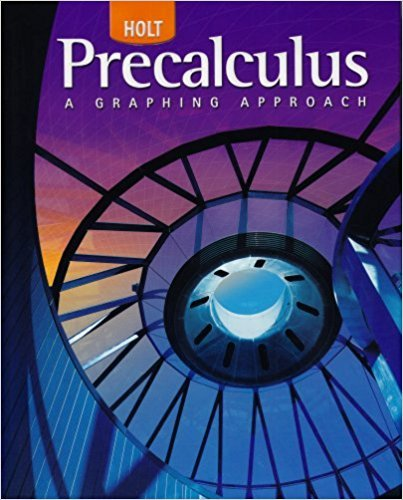×
×

# Solutions for Chapter 2.1: Solving Equations Graphically## Full solutions for Precalculus | 1st Edition

ISBN: 9780030416477Solutions for Chapter 2.1: Solving Equations Graphically

Solutions for Chapter 2.1
4 5 0 386 Reviews
23
4
##### ISBN: 9780030416477

Chapter 2.1: Solving Equations Graphically includes 44 full step-by-step solutions. Since 44 problems in chapter 2.1: Solving Equations Graphically have been answered, more than 24400 students have viewed full step-by-step solutions from this chapter. This expansive textbook survival guide covers the following chapters and their solutions. This textbook survival guide was created for the textbook: Precalculus, edition: 1. Precalculus was written by and is associated to the ISBN: 9780030416477.

Key Calculus Terms and definitions covered in this textbook
• Arctangent function

See Inverse tangent function.

• Cone

See Right circular cone.

• Coterminal angles

Two angles having the same initial side and the same terminal side

• equation of a hyperbola

(x - h)2 a2 - (y - k)2 b2 = 1 or (y - k)2 a2 - (x - h)2 b2 = 1

• Focal width of a parabola

The length of the chord through the focus and perpendicular to the axis.

• Imaginary unit

The complex number.

• Leibniz notation

The notation dy/dx for the derivative of ƒ.

• Linear regression

A procedure for finding the straight line that is the best fit for the data

• Magnitude of a real number

See Absolute value of a real number

• Mapping

A function viewed as a mapping of the elements of the domain onto the elements of the range

• Negative association

A relationship between two variables in which higher values of one variable are generally associated with lower values of the other variable.

• Outliers

Data items more than 1.5 times the IQR below the first quartile or above the third quartile.

• Parallel lines

Two lines that are both vertical or have equal slopes.

• Permutation

An arrangement of elements of a set, in which order is important.

• Right circular cone

The surface created when a line is rotated about a second line that intersects but is not perpendicular to the first line.

• Root of a number

See Principal nth root.

• Row operations

See Elementary row operations.

• Unit ratio

See Conversion factor.

• Vertex of an angle

See Angle.

• y-axis

Usually the vertical coordinate line in a Cartesian coordinate system with positive direction up, pp. 12, 629.

×